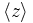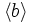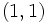# Subgroup structure of binary octahedral group

View subgroup structure of particular groups | View other specific information about binary octahedral group

This article discusses the subgroup structure of the binary octahedral group, which is given by the presentation:$\langle a,b,c \mid a^4 = b^3 = c^2 = abc \rangle$.

## Tables for quick information

FACTS TO CHECK AGAINST FOR SUBGROUP STRUCTURE: (finite group)
Lagrange's theorem (order of subgroup times index of subgroup equals order of whole group, so both divide it), |order of quotient group divides order of group (and equals index of corresponding normal subgroup)
Sylow subgroups exist, Sylow implies order-dominating, congruence condition on Sylow numbers|congruence condition on number of subgroups of given prime power order
normal Hall implies permutably complemented, Hall retract implies order-conjugate

### Quick summary

Item Value
Number of subgroups 35
Number of conjugacy classes of subgroups 13
Number of automorphism classes of subgroups 13
Isomorphism classes of Sylow subgroups and the corresponding Sylow numbers and fusion systems 2-Sylow: generalized quaternion group:Q16, Sylow number 3, fusion system ?
3-Sylow: cyclic group:Z3, Sylow number 4
maximal subgroups Maximal subgroups are: derived subgroup of binary octahedral group (isomorphic to SL(2,3), order 24), 2-Sylow subgroups of binary octahedral group (isomorphic to Q16, order 16), and subgroups of order 12 isomorphic to dicyclic group:Dic12

### Table classifying subgroups up to automorphisms

TABLE SORTING AND INTERPRETATION: Note that the subgroups in the table below are sorted based on the powers of the prime divisors of the order, first covering the smallest prime in ascending order of powers, then powers of the next prime, then products of powers of the first two primes, then the third prime, then products of powers of the first and third, second and third, and all three primes. The rationale is to cluster together subgroups with similar prime powers in their order. The subgroups are not sorted by the magnitude of the order. To sort that way, click the sorting button for the order column. Similarly you can sort by index or by number of subgroups of the automorphism class.
Automorphism class of subgroups Representative subgroup (full list if small, generating set if large) Isomorphism class Order of subgroups Index of subgroups Number of conjugacy classes (=1 iff automorph-conjugate subgroup) Size of each conjugacy class (=1 iff normal subgroup) Total number of subgroups (=1 iff characteristic subgroup) Quotient group (if it exists)
trivial subgroup$\{ e \}$ trivial group 1 48 1 1 1 binary octahedral group
center of binary octahedral group$\langle z \rangle$ cyclic group:Z2 2 24 1 1 1 symmetric group:S4
3-Sylow subgroups of binary octahedral group$\langle b^2 \rangle$ cyclic group:Z3 3 16 1 4 4 --
cyclic subgroups of order four$\langle c \rangle$ cyclic group:Z4 4 12 1 --
cyclic subgroups of order four$\langle a^2 \rangle$ cyclic group:Z4 4 12 1 --
cyclic subgroups of order six$\langle b \rangle$ cyclic group:Z6 6 8 1 4 4 --
quaternion subgroup of one type$\langle aca^{-3},c \rangle$ quaternion group 8 6 --

(remaining items to be transcribed into table later)

1. The quaternion group of the form PLACEHOLDER FOR INFORMATION TO BE FILLED IN: [SHOW MORE]. Isomorphic to quaternion group.
2. The cyclic groups conjugate to$\langle a \rangle$. Isomorphic to cyclic group:Z8. (3)
3. The dicyclic group of order$12$, i.e., the binary von Dyck group$\Gamma(3,2,2)$. Isomorphic to dicyclic group:Dic12. (4)
4. The generalized quaternion group of order$16$. Isomorphic to generalized quaternion group. (3)
5. A unique subgroup of order$24$, isomorphic to special linear group:SL(2,3). (1)
6. The whole group. (1)

### Table classifying isomorphism types of subgroups

Group name GAP ID Occurrences as subgroup Conjugacy classes of occurrence as subgroup Occurrences as normal subgroup Occurrences as characteristic subgroup
Trivial group$(1,1)$ 1 1 1 1
Cyclic group:Z2$(2,1)$ 1 1 1 1
Cyclic group:Z3$(3,1)$ 4 1 0 0
Cyclic group:Z4$(4,1)$ 9 2 0 0
Cyclic group:Z6$(6,2)$ 4 1 0 0
Quaternion group$(8,4)$ 4 2 1 1
Cyclic group:Z8$(8,1)$ 3 1 0 0
Dicyclic group:Dic12$(12,1)$ 4 1 0 0
Generalized quaternion group:Q16$(16,9)$ 3 1 0 0
Special linear group:SL(2,3)$(24,3)$ 1 1 1 1
Binary octahedral group$(48,28)$ 1 1 1 1
Total -- 35 13 5 5